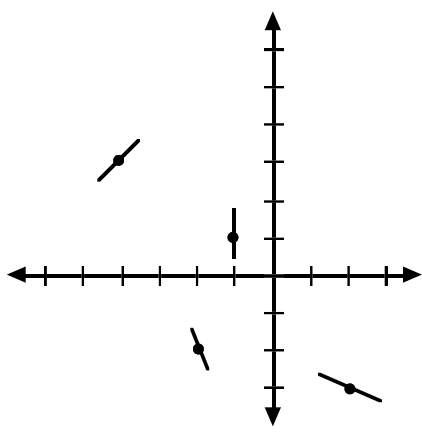### Home > CALC > Chapter 7 > Lesson 7.3.2 > Problem7-122

7-122.

Remember Theresa, the girl who loves tangents. Well, Theresa is at it again! She has drawn tangents to her function $g(x)$ and then erased the function.1. Trace her diagram on your paper. Using the tangents, sketch a possible function $g(x)$.

Make sure your sketch of $g(x)$ goes through all the coordinate points in order AND has the indicated slope at each point.

Recall that a local max or local min COULD exist in one of two places: where the derivative (slope) $= 0$ or where the derivative does not exist.
But, just because $g^\prime(x) = 0$ or $g^\prime(x) =$DNE, does not mean that that MUST be the location of a local max or local min.